# OECD Nuclear power plants data analysis 5 - Hypothesis test for One proportion using R infer package.UnsplashCraig Mannersが撮影した写真

This post is following of the above post.

In this post, I will do hypothesis test for one proportion. For Japan nuclear power plants proportion.

In the previous post, I found Japan nuclear power plants proportion is 0.111.

Now I set Null hypothesis as follows.
Null hypothesis: Japan nuclear power plants proportion is 0.16.

And Alternative hypothesis is as follows.

Alternative hypothesis: Japan nuclear power plants proportion is not 0.16.

I set alpha is 0.05( = 5%).

First, I make null distribution.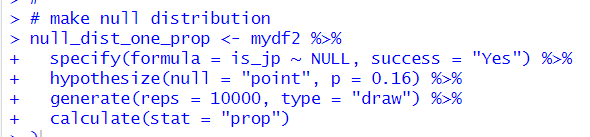Then, let's visualize this null distribution.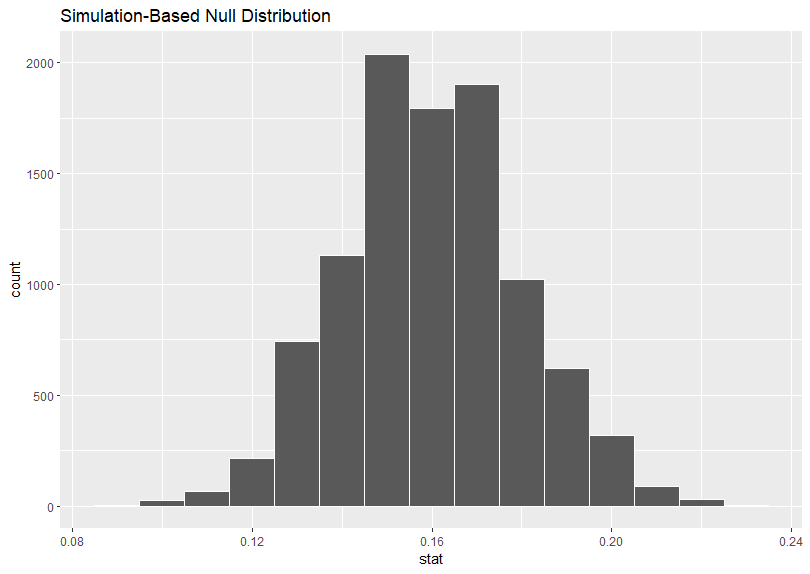Then, I visualize p-value.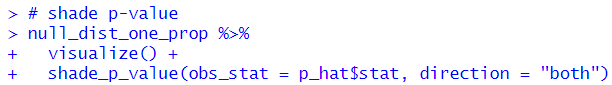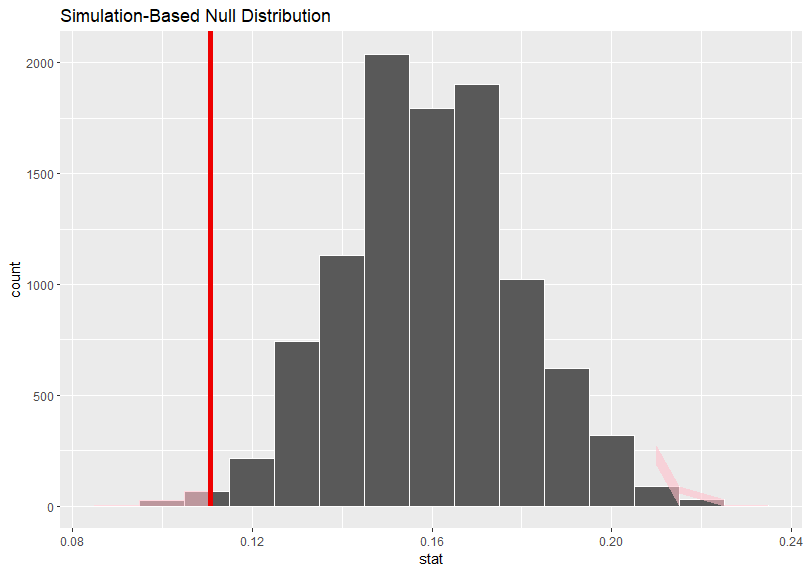Then, I use get_p_value() function to get p-value.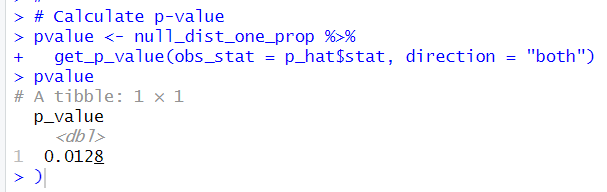P-value is 0.0128, which is smaller than alpha(0.05), thus I reject Null hypothesis.

I can say Japan nuclear power plants proportion is not 0.16.

prop_test() function gets traditional(formula based) p-value.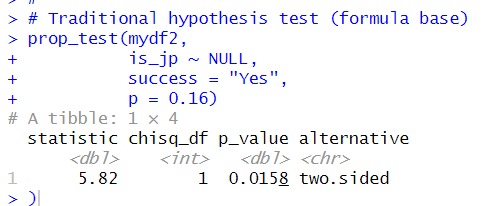I can also use prop.test() function.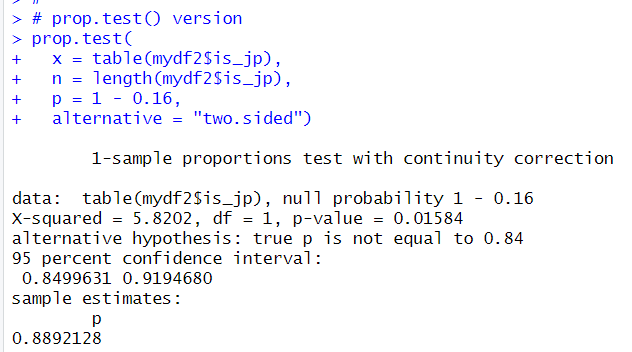p-value from prop.test() function is 0.01584, it is the same p-value from prop_test() function.

That's it. Thank you!